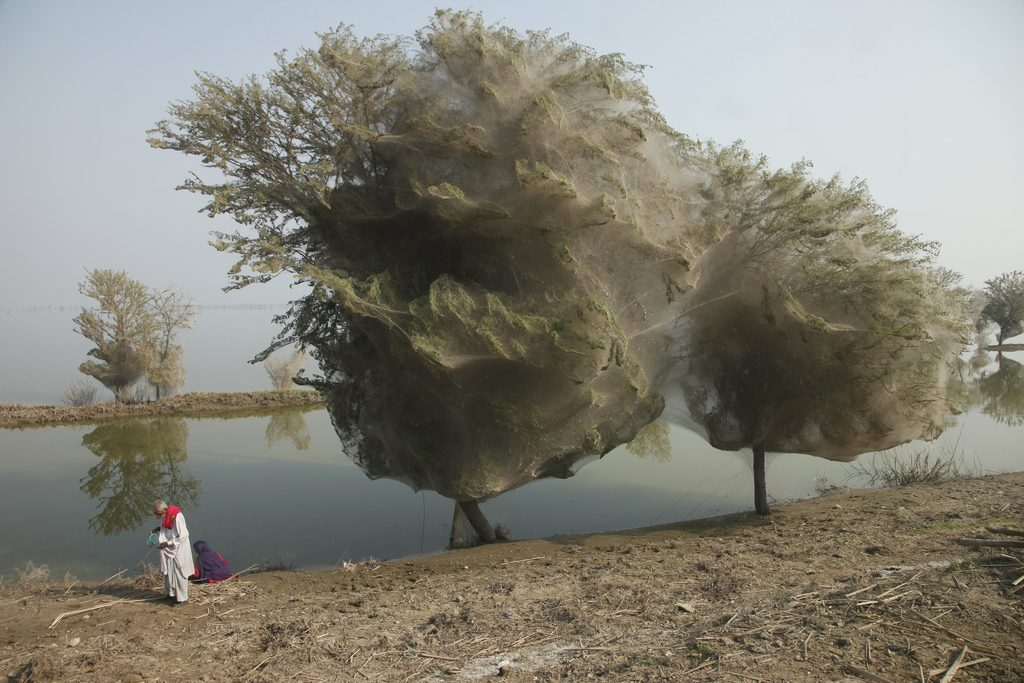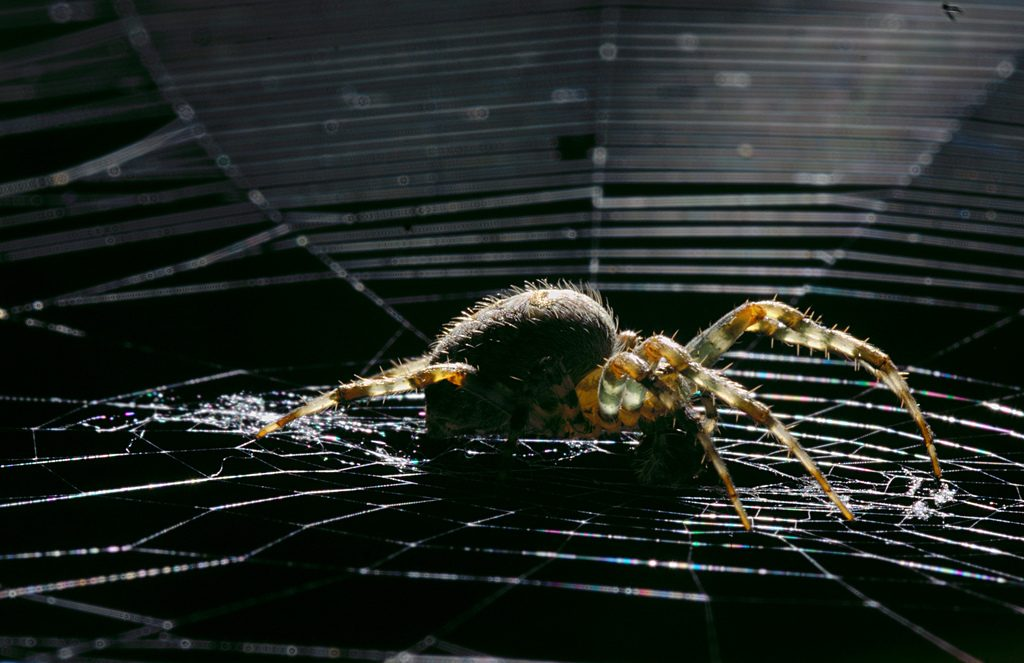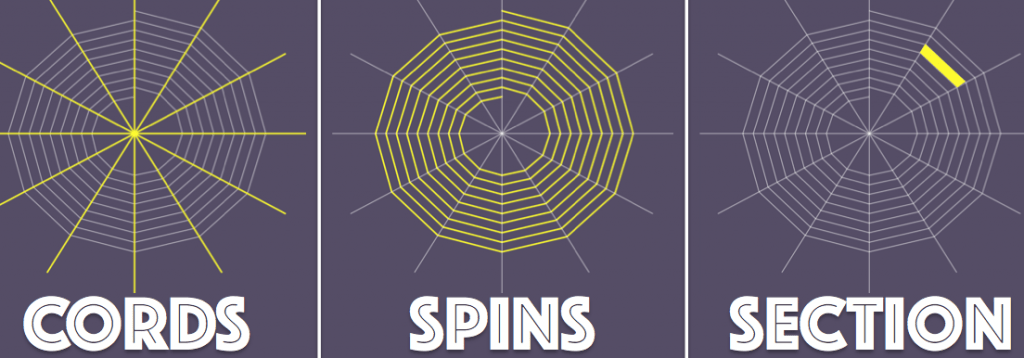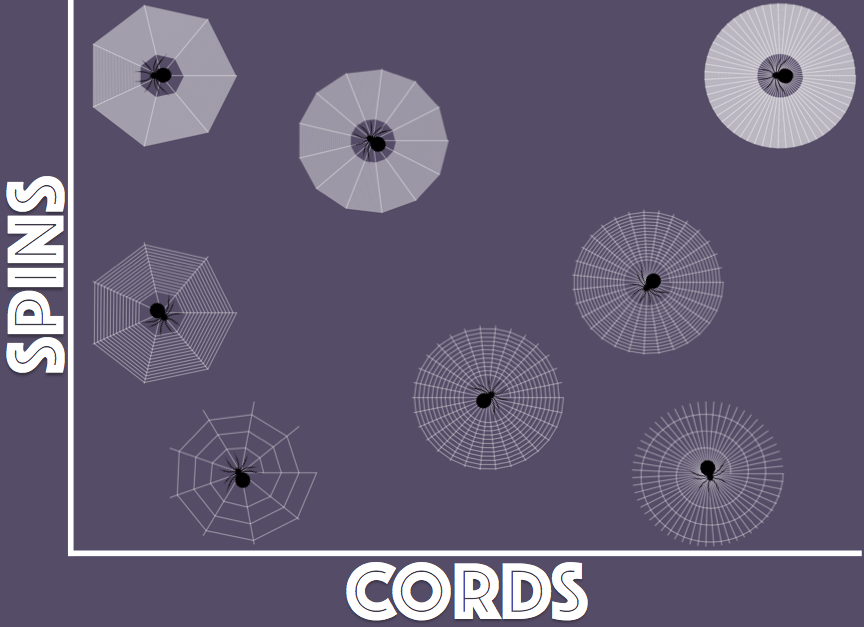The spider witch project

Constructing a spiderweb: in the spookiest and most horrific way possible!If spiders could count all the way up to forty, calculate angles and tensions, then perhaps we could explain why their webs all follow a similar pattern, having a similar number of strings and turns and flips… but the reality is that they (probably) can’t, so the fact that webs are similar must be because spiders are really good at minimising functions!Billions of spiders!

Firstly, do all spiders build similar webs? Not really. Some species of spiders don’t even construct webs and some of them make constructions that resemble funnels or tubes. Take the picture on the left, for example: would you ever like to walk near those trees? Not me! So, let’s only consider the type of spiderwebs that we can observe in a park or on a bench, and that look like a spiral orb, not like one we might find in some Alfred Hitchcock movie or a farm field in Pakistan.

In fact, all orbital webs actually look pretty much the same, whether spun by a Eriophora Transmarina from Australia or the Gasteracantha Cancriformis from Costa Rica. The point is that around the world there are more than 3,000 species of arachnids finding branches or the perfect corner in your house (apologies for the possible nightmares, but it is Halloween after all) to build basically the same structure.Spiders who build such webs, known as orb spiders, are the only terrestrial animal that feeds by filtering air (like some crustaceans and whales feed by filtering food particles from water). Their webs must, therefore, be designed to trap annoying mosquitoes and other, similarly annoying, insects so that you can sleep peacefully at night (you see: spiders are not that bad). Some spiders build a new web each day and some spiders even eat the old web and then spin a new web in the same location.Spiders are smart little animals and efficient as hell. They build a web incredibly quickly and aim to use as little resources (silk and time) as possible, while still making their web strong enough to support their weight, resist winds and storms and handle the hit of the prey, which is the lucky arachnid’s dinner. They start by using a not-so-sticky but super-strong silk from which they build two anchor points, forming a “V” from which they dangle down to make a starting “Y”. Further radial strands are then added from the centre of the “Y” to the edges of what will become the web; then a widely-spaced spiral is built from the centre outwards to strengthen the web; and then, this time with a super-sticky silk, they construct another, more closely spaced, spiral inwards. This is the one that traps the filthy insects that go by. Close enough to the centre of the web, they revert back to the non-sticky silk. since nobody likes living with a sticky floor, not even spiders!4 simple steps for creating your own spiderweb (if you are a spider, of course)

The spiderweb has a radius $R$ (OK, it’s not a perfect circle but let’s assume that it is) and they don’t use the sticky silk right in the centre, in a circle with radius $H$. We assume also that $R$ and $H$ are fixed and that they are determined by the size of the spider.A spiderweb with radius $R$ and hole $H$.

What the spider gets to control are the number of radial cords (let’s call it $c$) and the number of spins in the spiral ($s$). A lazy spider might decide to build the web with a small $c$, resulting in quite a fragile spiderweb; or it might decide to go for a small $s$, in which case the chances of the web catching prey are close to zero.Let’s assume that the time it takes for the spider to add an extra cord $c$ or an extra spin $s$ is the same. Although this might seem like a strong assumption (since a full spin is much larger than a cord), the addition of a cord is actually a rather complicated process, which takes longer than just spinning out the equivalent length of string. Therefore, the total time it takes for a spider to construct its web is given (in spider units of time) by

$$T(c, s) = c + s.$$

Spiders want to minimise the time invested in the construction of the web, $T$, but, at the same time, they want to make sure that any mosquitoes or flies that pass by get trapped: they need to ensure a delicious dinner! For this, they must construct the web such that the area of each of the sections in the diagram above is small enough to filter any bug that goes by.On each spiderweb there are (approximately) $c s$ sections, covering an area of (approximately) $\pi (R^2 – H^2)$, which means that the average size of each section of the spiderweb is given by

$$M(c, s) = \frac{\pi (R^2 – H^2)}{c s}.$$

Suppose that a spider is guaranteed a meal if $M(c, s) \leq \mu$, where $\mu$ is a certain number that depends on what the spider is trying to catch. Spiders are hungry! Therefore, spiders have a simple minimisation problem with restrictions:

$$\min T(c, s) = c + s \text{ subject to } M(c, s) = \frac{\pi (R^2 – H^2)}{c s} \leq \mu$$To ensure that the spiders has a delicious dinner, it needs to build its webs in the darker region. The red lines indicate different spiderwebs that require the same construction time, with a larger time in the upper-right corner of the graph.To solve this minimisation problem with restrictions, we can convert the restriction $M(c, s)\leq \mu$ into the equality $M(c, s) = \mu$ and so we can write

$$s =\frac{\pi (R^2 – H^2)}{ \mu c}.$$

In this way, it remains to minimise a function that depends on a single variable and has no restrictions:

$$T(c) = c +\frac{\pi (R^2 – H^2)}{ \mu c}.$$

Admittedly, we now have to differentiate a discrete number, but let’s remember that it’s Halloween and so witchcraft is perfectly valid. The solution gives us that the best spiderweb has $c^\star =s^\star$, the same number of spins and cords! and the number should be $c^\star =s^\star = \sqrt{\frac{\pi (R^2 – H^2)}{\mu}}$ depending on the dimension of the web they build, the hole for resting and also, the size of their lunch (the insects they try to catch).

Therefore, an average-size spider, which feeds mainly on stupid mosquitos and other annoying flies (not a whole mouse!), has nearly 31 cords and 31 spins. Perhaps, your favourite new pet!Yes, spiders are quite smart creatures, with webs surprisingly close to what we would consider to be a mathematical optimum! More complex models exist, considering, for example, the impact of the prey and its resistance. If you are not too scared of spiders, don’t miss this video from the BBC Earth Unplugged series (and only if you think you will never fear spiders, click here).